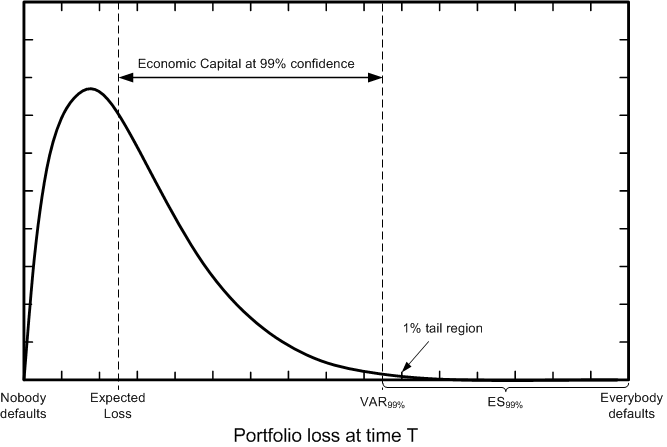# CCruncher

The Open-Source Project for Portfolio Credit Risk ModelingCCruncher-2.6.0 is a project for quantifying portfolio credit risk using the copula approach. CCruncher evaluates the portfolio credit risk by sampling the portfolio loss distribution and computing the Expected Loss (EL), Value at Risk (VaR) and Expected Shortfall (ES) statistics. The portfolio losses are obtained simulating the default times of obligors and simulating the EADs and LGDs of their assets. The solution consists of three components:

Keywords: portfolio credit risk modeling, copula approach, ratings, Probability of Default (PD), transition matrix, Exposure At Default (EAD), Loss Given Default (LGD), industrial sectors, sectorial correlation, multi-factor model, Gaussian copula, t-Student copula, Monte Carlo, MCMC, Expected Loss (EL), Value at Risk (VaR), Expected Shortfall (ES), risk sensitivity, portfolio optimization, open-source.

##### Technical Document
The Technical Document provides a comprehensible view of portfolio credit risk modeling using the copula approach (t-Student case). It emphasizes the practical aspects, including short introductions of methods, examples, and a discussion of the implementation details.
##### Parameter Estimation
CCruncher-inf is a software program that estimates the dependency parameters (correlations and degree of freedom) of the multi-factor model based on historical data (number of defaults per year-sector-rating). The estimates are made using the Bayesian approach through MCMC.
##### Risk Estimation
CCruncher-gui and CCruncher-cmd are software programs that simulate the loss distribution of a credit portfolio using the Monte Carlo method. Risk measures (EL, VaR, ES) can be obtained computing the usual estimators (mean and percentile) with your favorite data analysis tool.